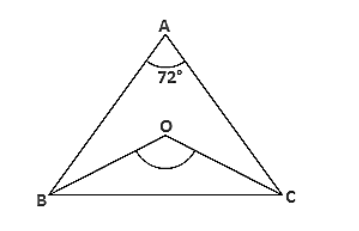# ABC is a triangle in which ∠A = 720°, the internal bisectors of angles B and C meet in O.

Question:

ABC is a triangle in which ∠A = 720°, the internal bisectors of angles B and C meet in O. Find the magnitude of ∠BOC.Solution:

Given,

ABC is a triangle where ∠A = 72° and the internal bisector of angles B and C meeting O.

In ΔABC,

∠A + ∠B + ∠C = 180°

⇒ 72° + ∠B + ∠C = 180°

⇒ ∠B + ∠C = 180° − 72°

Dividing both sides by '2'

⇒ ∠B/2 + ∠C/2 = 108°/2

⇒ ∠OBC + ∠OCB = 54°

Now, In ΔBOC ⇒ ∠OBC + ∠OCB + ∠BOC = 180°

⇒ 540° + ∠BOC = 180°

⇒ ∠BOC = 180° − 54°=126°

∴  ∠BOC = 126°